### Simplicity Functions for Rotations

To rotate a factor pattern is to apply a nonsingular linear transformation to the unrotated factor pattern matrix. An optimal transformation is usually defined as a minimum or maximum point of a simplicity function. Different rotation methods are based on different simplicity functions employed.

For the promax or the Procrustes rotation, the simplicity function used is the sum of squared differences between the rotated factor pattern and the target matrix. The optimal transformation is obtained by minimizing this simplicity function with respect to the choices of all possible transformation.

For the class of the generalized Crawford-Ferguson family Jennrich (1973), the simplicity function being optimized is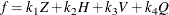where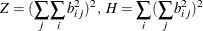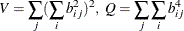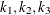, and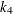are constants, and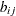represents an element of the rotated pattern matrix. Except for specialized research purposes, it is uncommon in practice to use this simplicity function directly for rotation. However, this simplicity function reduces to many well-known classes of rotations. One of these is the Crawford-Ferguson family Crawford and Ferguson (1970), which minimizes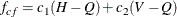where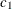and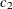are constants,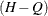represents variable (row) parsimony, and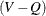represents factor (column) parsimony. Therefore, the relative importance of both the variable parsimony and of the factor parsimony is adjusted using the constantsand. The orthomax class (see Harman 1976) maximizes the function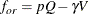whereis the orthomax weight and is usually between 0 and the number of variables p. The oblimin class minimizes the function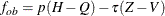whereis the oblimin weight. For practical purposes, a negative or zero value foris recommended.

All of the preceding definitions are for rotations without row normalization. For rotations with Kaiser normalization, the definition ofis replaced by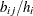, where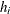is the communality estimate of variable i.